• 数据可视化函数plt.plot(x,y,ls,lw,c,marker,markersize,markeredgecolor,markerfacecolor,label) 设置画线的标准和形式 参数说明： **x：**横坐标；**y：**纵坐标；**ls或linestyle：**线的形式（‘-’，‘–’，...

参考：（下图来自）https://blog.csdn.net/qiurisiyu2016/article/details/80187177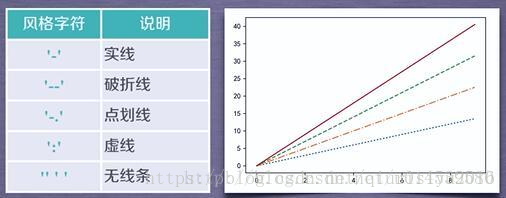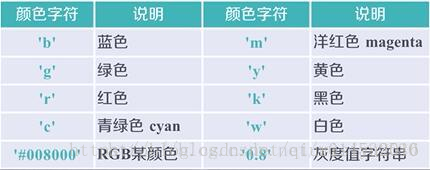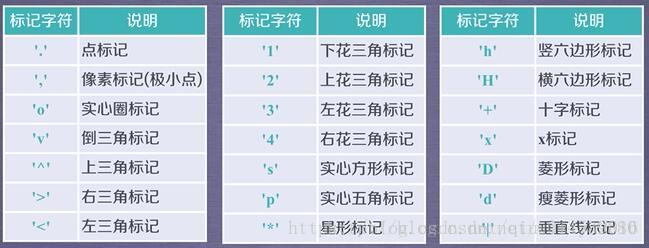数据可视化函数plt.plot(x,y,ls,lw,c,marker,markersize,markeredgecolor,markerfacecolor,label)

设置画线的标准和形式
参数说明：
**x：**横坐标；**y：**纵坐标；**ls或linestyle：**线的形式（‘-’，‘–’，‘：’和‘-.’）；**lw（或linewidth）：**线的宽度；**c：**线的颜色；**marker：**线上点的形状；**markersize或者ms：**标记的尺寸，浮点型；**markerfacecolor：**点的填充色；**markeredgecolor：标记的边沿颜色label：**文本标签

import numpy as np
import pandas as pd
import matplotlib.pyplot as plt

#使能够正常显示中文
plt.rcParams['font.sans-serif']=['SimHei']
plt.rcParams['axes.unicode_minus']=False

x=np.linspace(0,360,20) #建立20个从0到哦360的线性平均数据
y=np.sin(x/180*3.1415926)	#将x的角度值转换成弧度值
y1=np.sin(x/180*3.1415926)-2	#将x对应的y值整体向下平移2
plt.plot(x,y,c='red',lw=2,ls='-') #第一个红线：线宽为2，线性‘-’
#下面黑线，红点、黄轮廓的线
plt.plot(x,y1,c='black',lw=4,ls='-.',marker='o',\
markersize=8,markerfacecolor='red',markeredgecolor='yellow',label='x和y对应的点和线')
plt.legend(loc='center') #显示标签位置
plt.show()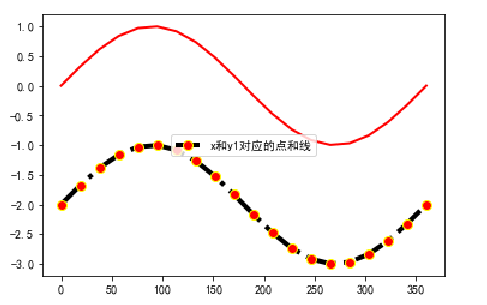调整图片的参数plt.figure（figsize=( ),dpi=,facecolor=）

**dpi:**分辨率
**facecolor：**图片背景色

y轴刻度plt.yticks( )

import numpy as np
import pandas as pd
import matplotlib.pyplot as plt

#使能够正常显示中文
plt.rcParams['font.sans-serif']=['SimHei']
plt.rcParams['axes.unicode_minus']=False
x=np.linspace(0,720,40)
y=np.sin(x/180*3.1415926)-2
y1=np.sin(x/180*3.1415926)

plt.figure(figsize=[12.5,8.84],dpi=100,facecolor='orange')
plt.title('这是整幅图的标题')
plt.xlabel('x轴')
plt.ylabel('y轴')
plt.xticks(np.linspace(0,720,21))		#这里想要20段，但必须有21个标签
#plt.xlim([0,360])  未限制x轴显示
plt.plot(x,y1,c='black',lw=4,ls='-.',marker='o',\
markersize=8,markerfacecolor='red',markeredgecolor='yellow',label='x和y1对应的点和线')
plt.legend(loc=0,fontsize='large',title='这是一个标题')
plt.show()	#第一次显示图片
plt.figure(figsize=[12.5,8.84],dpi=100,facecolor='orange')
plt.xticks(np.linspace(0,720,21))
plt.xlim([0,720])
plt.plot(x,y1,c='black',lw=4,ls='-.',marker='o',\
markersize=8,markerfacecolor='red',markeredgecolor='yellow',label='x和y1对应的点和线')
plt.show()  #第二次显示图片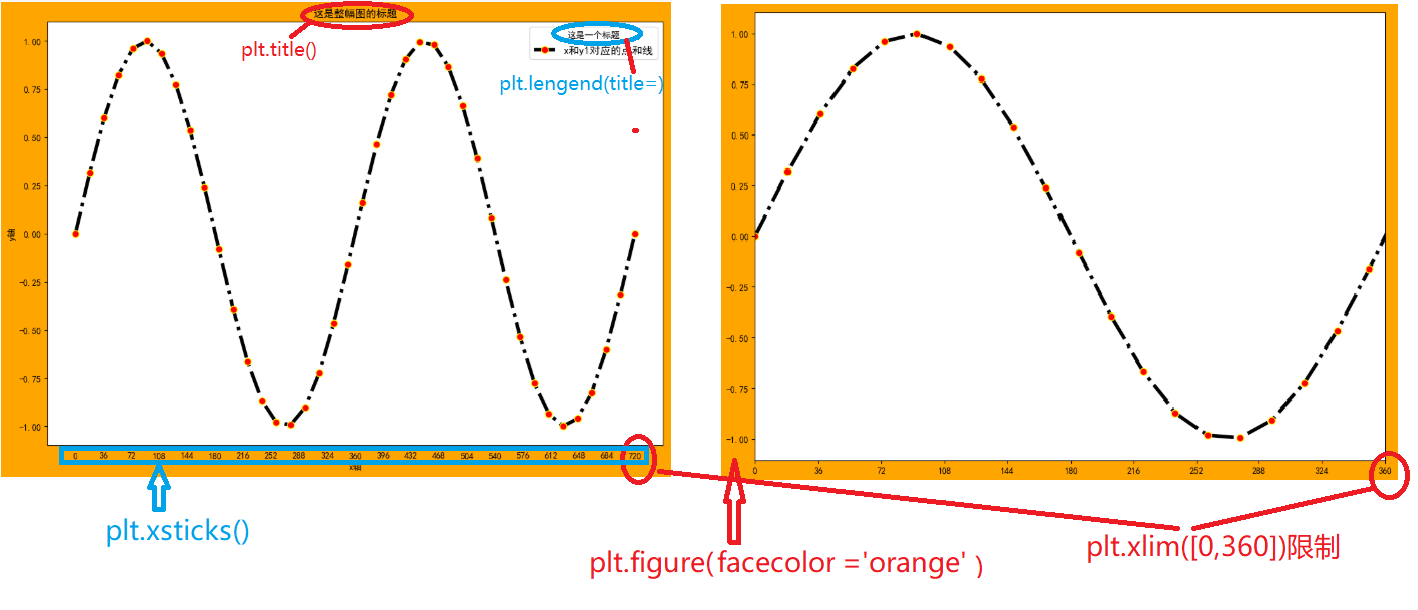保存plt.savefig(‘路径和名称’)

需要保存的图片在plt.show()上一句使用该命令

展开全文python plt.plot
• 可自动排序，最后只需使用plt.bar(tick_label=[ ])修改横坐标的标签字符即可 2、建立直方图时，使用的数据是自己处理后的变量索引和column，而且处理数据一般使用np.sum(),记录一类数量。 3、为了显示的图形不会...

柱状图plt.bar

同一柱状图堆叠显示plt.pivot()+plt.bar(bottom=)

对于不同季度，不同产品的质量评价显示，先将使用的数据内容通过pd.pivot_table()进行数据筛选，重新设置了索引列和列标题（columns），再对数据使用柱状图显示

import numpy as np
import pandas as pd
import matplotlib.pyplot as plt

print(data)
#解决中文乱码问题
plt.rcParams['font.sans-serif']=['SimHei']
plt.rcParams['axes.unicode_minus']=False
plt.figure(figsize=(6.4,4.8))#设置图片背景的参数
print('下面是整理后的数据：==========')
#通过pivot_table函数重新提取数据两列作为(data)索引列和横向的列名（columns），另外的vlues对应的是数列,注意的是，尽量让索引是和数字有关的序列，可自动排序
over_view=pd.pivot_table(data=data,index='Q',columns='GOODS',values='QUA',aggfunc=np.sum)
print(over_view)
#使用tick_label对x轴的标签重新赋值
plt.bar(x= over_view.index.values,height=over_view.,color='green',tick_label=['第一季度','第二季度','第三季度','第四季度'] )
plt.bar(x= over_view.index.values,height=over_view.,bottom=over_view.,color='red')
plt.bar(x= over_view.index.values,height=over_view.,bottom=over_view.+over_view.,color='orange')
plt.xticks(rotation=45)	#x轴上的标签旋转45度
plt.show()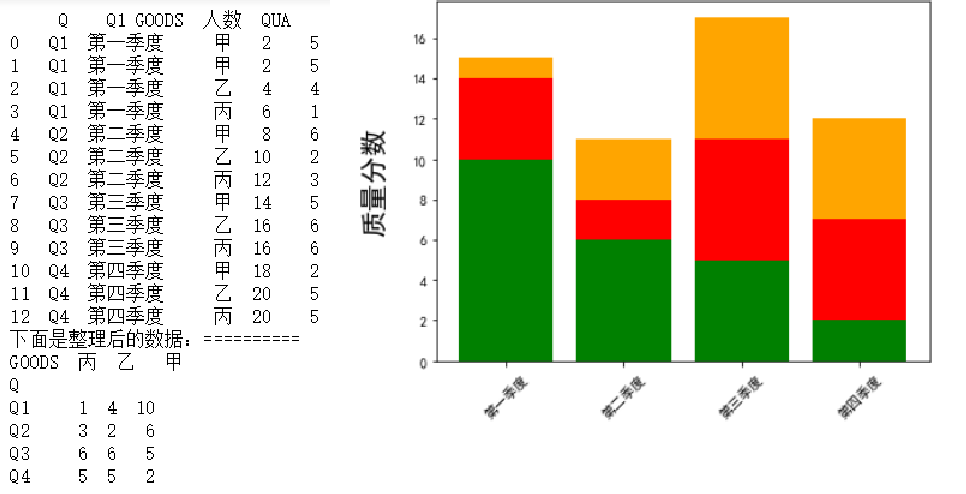通过上边的程序和运行结果，得出一下几点注意，
1、索引尽量使用能够数字排序的字符，可自动排序，到最后只需使用plt.bar(tick_label=[ ])修改横坐标的标签字符即可
2、建立直方图时，使用的数据是自己处理后的变量索引和column，而且处理数据一般使用np.sum(),记录一类数量。
3、为了显示的图形不会出现遮挡，需要使用到plt.bar(bottom=),将想要放在下边的数据作为值传入，若不使用，每次图形的最低端都是以横轴开始计算，若出现完全遮挡，则图形无意义
上例中，每个季度，三个产品的服务质量评分。让季度和物品名称（甲乙丙）作为横纵参数，服务质量分数作为数据显示

同一柱状图堆叠显示plt.crosstab(nomalize=)+plt.bar(bottom=)

堆叠显示，对数据选择并单位化，可以明显看出，每个阶段数据量占的百分比
而且再使用bar绘制图形时，要设置label属性的值，否则，使用plt.legend时会出现问题
plt.legend（）的显示位置不合适，可以通过plt.legend()中的bbox_to_anchor=(坐标)，认为图形的右上角是（1，1），可设置（1.01，0.8）将内容注释放在图像外部

#前面和上个程序一样
over_view=pd.crosstab(data.Q,data.GOODS,normalize='index', values=data.QUA,aggfunc=np.sum,)
print(over_view)
plt.bar(x= over_view.index.values,height=over_view.,color='green',label='甲',tick_label=['第一','第二','第三','第四'] )
plt.bar(x= over_view.index.values,height=over_view.,bottom=over_view.,label='乙',color='red')
plt.bar(x= over_view.index.values,height=over_view.,label='丙',bottom=over_view.+over_view.,color='orange')
plt.xticks(rotation=45)#旋转横坐标标签
plt.legend(bbox_to_anchor=(1.01,0.8))
plt.show()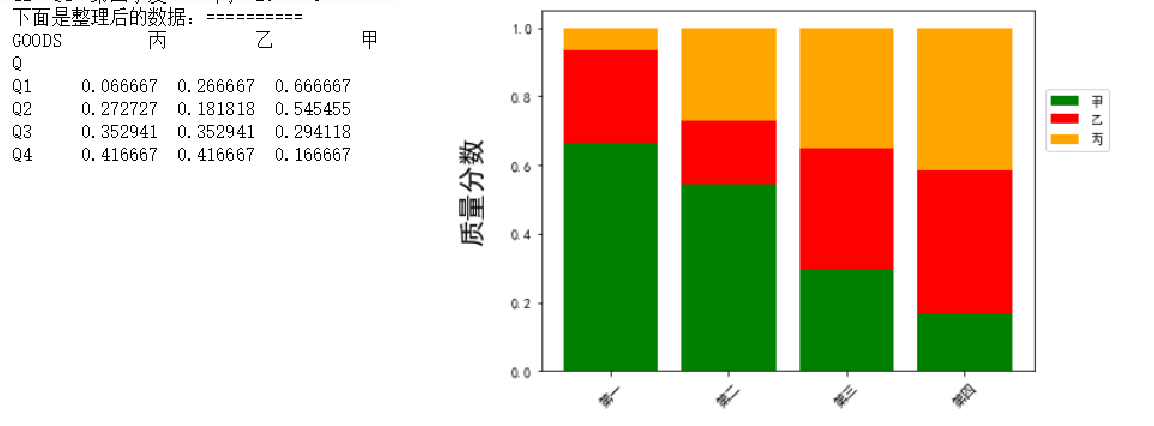程序解析：这个程序及绘制的图形是通过pd.crosstab( )将数据的每一行（也就是index的每行）归一化

直方图plt.hist

正态分布函数，下面函数返回对应的计算正态频率值输出

def zhengtai_func(x,miu,sigma):
zhen_y=np.exp(-(x-miu)**2/(2*(sigma**2)))/(sigma*np.sqrt(2*np.pi))
return zhen_y

正态计算公式；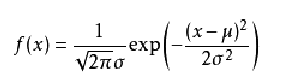print(data)
#解决中文乱码问题
plt.rcParams['font.sans-serif']=['SimHei']
plt.rcParams['axes.unicode_minus']=False

#删除数据中缺失项，计算数据的均值，标准差，和正态分布频率点
data.dropna(subset=['人数'],inplace=True)
print(data)
#x_mean=np.mean(data.人数) #与下一行数据功能相同
x_mean=data.人数.mean()
x_std=np.std(data.人数)
x=np.arange(data.人数.min(),data.人数.max(),0.1)
y=zhengtai_func(x,x_mean,x_std)

#显示直方图，核密度图，设置注释标签label
plt.hist(x=data.人数,bins=5,color='lightblue',label='年龄频率',\
edgecolor='orange',density=True)
plt.plot(x,y,color='red',linewidth=3,label='正态分布线')
data.人数.plot(kind='kde',color="black",xlim=[0,10],label='核密度图')
plt.legend(loc='best')
plt.show()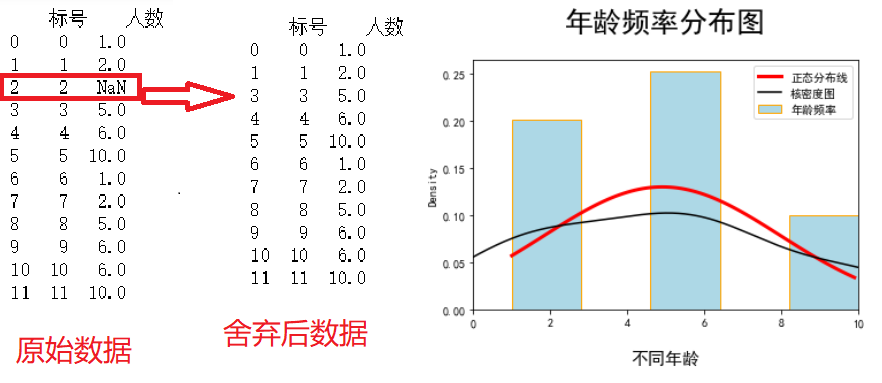程序及图形解析：
1、先定义了正态分布的概率计算，参数分别是，数据x,平均值x_mean,标准差x_std，返回计算的概率值，
2、然后读取数据，data.dropna( )删除缺失数据行，计算数据均值，标准差，取多个x值，通过正态公式显示成线，（使用数据的平均值和标准差就确定了数据的正态概率分布图）。
3、绘制直方图plt.hist( )，正态分布线plt.plot( x,y),核密度分布线data.plot(kind=‘kde’)、
4、核密度分布和正态分布区别：核密度分布和数据的每阶段的关系更大，正态分布确定了平均平均值和标准差后就确定了

箱图plt.boxplot()

参考：箱体知识参数知识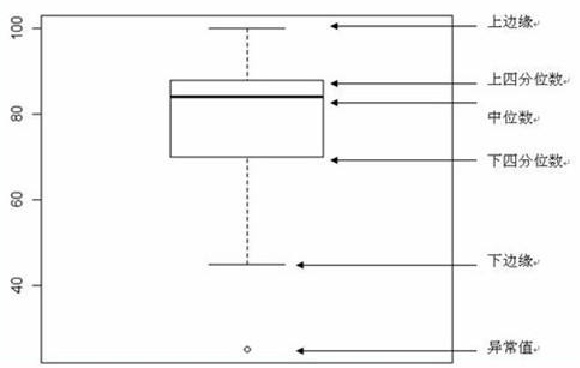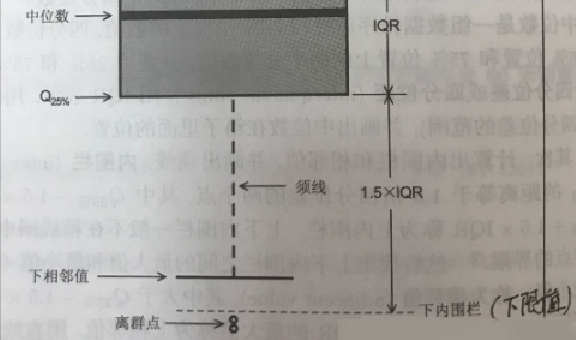plt.boxplot(x, notch=None, sym=None, vert=None, whis=None, positions=None, widths=None, patch_artist=None, bootstrap=None, usermedians=None, conf_intervals=None, meanline=None, showmeans=None, showcaps=None, showbox=None, showfliers=None, boxprops=None, labels=None, flierprops=None, medianprops=None, meanprops=None, capprops=None, whiskerprops=None, manage_xticks=True, autorange=False, zorder=None, hold=None, data=None)
**x：**指定要绘制箱线图的数据；
notch：是否是凹口的形式展现箱线图，默认非凹口；
sym：指定异常点的形状，默认为+号显示；
vert：是否需要将箱线图垂直摆放，默认垂直摆放；
whis：指定上下须与上下四分位的距离，默认为1.5倍的四分位差；
positions：指定箱线图的位置，默认为[0,1,2…]；
widths：指定箱线图的宽度，默认为0.5；
**patch_artist：**是否填充箱体的颜色；
**meanline：**是否用线的形式表示均值，默认用点来表示；
**showmeans：**是否显示均值，默认不显示；
showcaps：是否显示箱线图顶端和末端的两条线，默认显示；
**showbox：**是否显示箱线图的箱体，默认显示；
showfliers：是否显示异常值，默认显示；
**boxprops：**设置箱体的属性，如边框色，填充色等；
labels：为箱线图添加标签，类似于图例的作用；
filerprops：设置异常值的属性，如异常点的形状、大小、填充色等；
**medianprops：**设置中位数的属性，如线的类型、粗细等；
**meanprops：**设置均值的属性，如点的大小、颜色等；
**capprops：**设置箱线图顶端和末端线条的属性，如颜色、粗细等；
whiskerprops：设置须的属性，如颜色、粗细、线的类型等；

print(data)
#解决中文乱码问题
plt.rcParams['font.sans-serif']=['SimHei']
plt.rcParams['axes.unicode_minus']=False

plt.boxplot(x=data.人数,patch_artist=True,showmeans=True,meanline=True, boxprops={'facecolor':'green','color':'red'},\
flierprops={'marker':'o','markerfacecolor':'red','markersize':20},\
medianprops={'linestyle':'--','color':'orange'},\
meanprops={'linestyle':'-','color':'blue'})
# plt.legend(loc='best')
plt.show()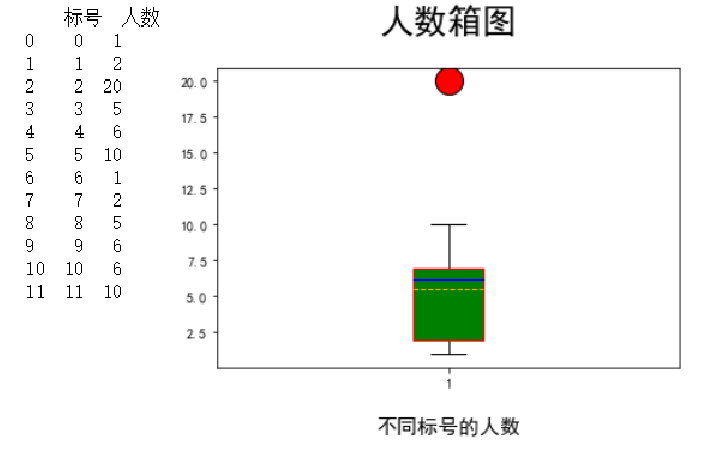程序解释及图形描述，箱图能够明显的显示出离群点，箱子的显示框在分位数的25%-75%，下线：25%分数-1.5(75%分位数-25%分位数)，上线：25%分数+1.5(75%分位数-25%分位数)，

散点图plt.scatter

感谢鸢尾花数据
鸢尾花数据官方网址,下载或打开慢
为了展示两个连续型变量的关系，使用循环为每一个事物给不同的显示属性，注意数据的名称准确性

# print(data)
#解决中文乱码问题
plt.rcParams['font.sans-serif']=['SimHei']
plt.rcParams['axes.unicode_minus']=False

#设置不同物种的颜色形状标记
species=['virginica','setosa','versicolor']
colors=['red','blue','orange']
marker=['o','s','x']

#给每个物种不同标记
for i in range(0,3):
plt.scatter(x=data.Width[data.Species==species[i]],
y=data.Length[data.Species==species[i]],
color=colors[i],marker=marker[i],label=species[i])
plt.legend(loc='best')

plt.show()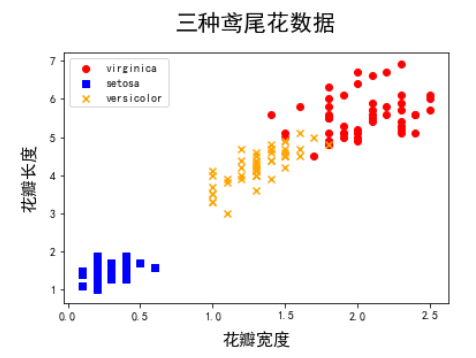折线图plt.plot()

显示数据的变化趋势

#设置图像大小和读取数据
fig=plt.figure(figsize=(8,7))
print(data)

#解决中文乱码问题
plt.rcParams['font.sans-serif']=['SimHei']
plt.rcParams['axes.unicode_minus']=False

#设置第1列作为x的值，第3，4，5列作为对应的y值
plt.plot(data.iloc[:,0],data.iloc[:,2],'bs--',
data.iloc[:,0],data.iloc[:,3],'ro--',
data.iloc[:,0],data.iloc[:,4],'gh--',)

#修改折线图的横坐标名称，第一个参数是原始名称，第二个参数是新的名称
plt.xticks(range(0,12,1),data.iloc[range(0,12,1),1],rotation=45,fontsize=10)
plt.legend(loc='best')

plt.show()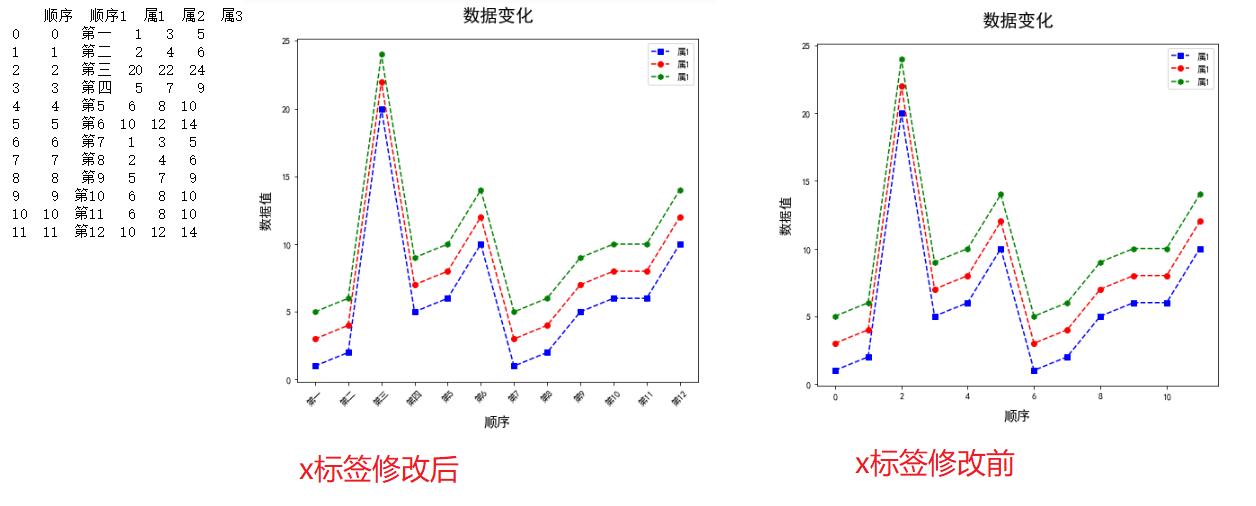下面是对图像中线的描述，（程序和图像并不对应），图片中的参数正确

plt.legend(loc='best',frameon=False,ncol=1)
plt.legend(loc='best',frameon=True,ncol=1)
plt.legend(loc='best',frameon=False,ncol=3)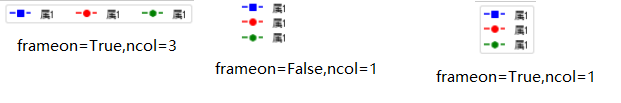展开全文• matplotlib ...num=1 设置图表序号，默认从一开始 figsize(20,8) 设置画布的宽高 dpi=80 设置图表的dpi plt.xticks，plt.yticks设置x轴y轴的刻度 设置x轴y轴的刻度 plt.xticks(x,name) plt.yticks([-2,

matplotlib

matplotlib是python底层绘图库，主要做数据可视化图表，模仿MATLAB构建

默认不支持中文字符，因为默认的英文字体无法显示中文
支持中文（matplotlib.rcParams['font.sans-serif'] = ['SimHei']）

在绘图时，先绘线，后设置其他，否则可能被绘线覆盖原设置

plt.figure修改图表大小

修改图表大小
plt.figure(num,figsize,dpi)
num=1    设置图表序号，默认从一开始
figsize(20,8)    设置画布的宽高
dpi=80    设置图表的dpi

plt.title设置图表标题

plt.title(str)
plt.title('10点-12点的温度变化情况')    设置图表标题

plt.grid绘制网格

绘制网格
plt.grid(alpha)
alpha=0.5    alpha为设置网格线条，值为0-1

plt.xticks，plt.yticks设置x轴y轴的刻度

设置x轴y轴的刻度
plt.xticks(x,name,rotation)
plt.yticks([-2, -1, 0, 1, 2],
[good, good1, good2, good3])
x为x轴的点(可以为数组)
name为字符串列表
rotation为name旋转的度数
y同上

plt.xlim，plt.ylim设置x轴y轴显示的范围

设置x轴y轴显示的范围
plt.xlim(-1,1)
plt.ylim(-1,1)

plt.xlabel，plt.ylabel设置x轴y轴的描述信息

设置x轴y轴的描述信息
plt.xlabel(str,rotation)
plt.ylabel('i am y',rotation=90)
plt.xlabel('i am y')    str为描述信息
rotation=90    rotation为str旋转的度数
plt.ylabel同上

plt.plot在图表中绘制一条线

在图表中绘制一条线
plt.plot(x, y,color,linewidth,linestyle,label,alpha)
x,y    x为x轴的点(可以为数组),y为y轴的点(可以为数组)
color='blue'    color为线条颜色默认蓝色（r红色，g绿色，b蓝色，w白色，c青色，m洋红，
y黄色，k黑色，也可传入十六进制#000000）
linewidth=1.0    linewidth为线的粗细
linestyle='--'    linestyle为线的样式（-实线，--虚线，-.点划线，:点虚线，' '留空或空格）
label='自己'    label为线的图例名
alpha=0.5    alpha为线条透明度

plt.scatter绘制散点图

在图表中绘制散点图
plt.scatter(x,y,label,color,alpha)
x,y    x为x轴的点(可以为数组),y为y轴的点(可以为数组)
color='blue'    color为线条颜色默认蓝色（r红色，g绿色，b蓝色，w白色，c青色，m洋红，
y黄色，k黑色，也可传入十六进制#000000）
label='自己'    label为线的图例名
alpha=0.5    alpha为线条透明度

plt.bar绘制柱状图（条形图）

绘制条形图
plt.bar(x,y,width,label)
x,y    x为横坐标，y为纵坐标
width    width为条形的粗细
label    laber为图例名

plt.barh绘制横向柱状图（条形图）

绘制条形图
plt.barh(x,y,height,color)
x,y    x为纵坐标，y为横坐标
height=0.5    height为条形的粗细
color='orange'    color为条形的颜色

plt.hist绘制直方图

绘制直方图
plt.hist(x,num)
x    x为数据列表
num    num为直方图分组数

plt.legend显示图例

显示图例
plt.legend(handles,label,loc)
handles=[l1,l2]    handles为线条的实例化对象
label=['aaa','bbb']    label为线条的图例名
loc='best'    loc为图例框显示在图中的位置（best为自动寻找合适位置，upper right为右上
upper left为左上，lower left为左下，lower right为右下，right为右，center left为中左
center right为中右，lower center为底部居中，upper center为顶部居中，center为全局居中）

plt.savefig保存图表

保存图表
plt.savefig('h.png')

展开全文开发语言 后端
• 例子1：控制图的X坐标和Y坐标的取值范围 方法1plt.axis([xmin，xmax，ymin，ymax]) 方法2：plt.xlim(xmin, xmax), plt.ylim(ymin, ymax) 例子2：控制图x，y坐标的刻度 plt.xticks(), plt.yticks() 例子3：控制...

Python - Matplotlib

Matplotlib 是一个用于在 Python 中绘制数组的图形库
下面代码可以直接在python环境下运行

目录

例子1：控制图的X坐标和Y坐标的取值范围

• 方法1：plt.axis([xmin，xmax，ymin，ymax])
• 方法2：plt.xlim(xmin, xmax), plt.ylim(ymin, ymax)

例子2：控制图x，y坐标的刻度

• plt.xticks(), plt.yticks()

例子3：控制图的图例

• plt.plot(x, y, label)
• plt.legend(), 它的labels参数控制labels, loc 参数控制图例位置

例子4：控制图的注释

• plt.annotate(text, xy, xytext, arrowprops)

正文

例子1：控制图的X坐标和Y坐标

import random
import numpy as np
#随机产生20个1-50/30/20之间的整数(不包含50，30，20)
y1 = np.random.randint(1,50,20)
y2 = np.random.randint(1,30,20)
y3 = np.random.randint(1,15,20)

x = np.arange(20)

#plot 结果是下面图1
import matplotlib.pyplot as plt

#在一个图里面展示三条线
plt.plot(x, y1, '--')
plt.plot(x, y2, '--')
plt.plot(x, y3, '--')

#控制图的x,y坐标轴的取值范围
plt.axis([-1,20,0,80])
plt.show()

#测试plt.xlim 和 plt.ylim， 结果如图2
import matplotlib.pyplot as plt

#在一个图里面展示三条线
plt.plot(x, y1, '--')
plt.plot(x, y2, '--')
plt.plot(x, y3, '--')

#控制图的x,y坐标轴的取值范围
plt.xlim(-1,30)
plt.ylim(0,70)
plt.show()

上面代码生成的图：例子2：控制图x，y坐标的刻度

• 使用上面的代码中的x, y1, y2, y3值
• plt.xticks(), plt.yticks()
#测试plt.xlim 和 plt.ylim
import matplotlib.pyplot as plt

#在一个图里面展示三条线
plt.plot(x, y1, '--')
plt.plot(x, y2, '--')
plt.plot(x, y3, '--')

#控制图的x,y坐标轴的数值
y_axis = np.arange(0,50,5)
plt.xticks(x)
plt.yticks(y_axis)
plt.show()

结果：
这时候x轴的坐标变成了0-19间距为1， y轴变成了0-50间距为5例子3：控制图的图例

• plt.legend(), 控制label的显示，注释掉，则label内容不显示。
#测试labels 和 plt.legend()
import matplotlib.pyplot as plt

#在一个图里面展示三条线
plt.plot(x, y1, '--', label='Y1: Mean=%.2f'%np.mean(y1))
plt.plot(x, y2, '--', label='Y2: Mean=%.2f'%np.mean(y2))
plt.plot(x, y3, '--', label='Y2: Mean=%.2f'%np.mean(y2))

#控制图的x,y坐标轴的数值
y_axis = np.arange(0,50,5)
plt.xticks(x)
plt.yticks(y_axis)

#控制图的显示
plt.legend()
plt.show()

• 另一种控制label展示的方式， plt.legend()。
• plt.legend(loc=“lower left”), 它包含loc 函数，可以控制图例的位置。
• 官方文档
#测试label在plt.legend()的另一种显示方式
import matplotlib.pyplot as plt

#在一个图里面展示三条线
plt.plot(x, y1, '--')
plt.plot(x, y2, '--')
plt.plot(x, y3, '--')

#控制图的x,y坐标轴的数值
y_axis = np.arange(0,50,5)
plt.xticks(x)
plt.yticks(y_axis)

#控制图的显示， 把图例显示在做下角
plt.legend(labels=('Y1: Mean=%.2f'%np.mean(y1),'Y2: Mean=%.2f'%np.mean(y2),'Y2: Mean=%.2f'%np.mean(y2)), loc='lower left')
plt.show()

结果例子4：控制图的注释

• 官方文档
• plt.annotate(text, xy, xytext), 不附加xytext，注释显示在点附近
• 参数：
• text：注释的内容
• xy：要注释的点
• xytext： 注释文本显示的位置
#测试plt.annotate
import matplotlib.pyplot as plt

#在一个图里面展示三条线
plt.plot(x, y1, '--', label='Y1: Mean=%.2f'%np.mean(y1))
plt.plot(x, y2, '--', label='Y2: Mean=%.2f'%np.mean(y2))
plt.plot(x, y3, '--', label='Y2: Mean=%.2f'%np.mean(y2))

#控制图的x,y坐标轴的数值
y_axis = np.arange(0,50,5)
plt.xticks(x)
plt.yticks(y_axis)

#控制图的注释
plt.annotate('The Max value is %d'%max(y1), #控制注释的内容
xy=(y1.index(max(y1)),max(y1)) #要注释的点，y1.index(max(y1)):y1中最大值的索引， max(y1)：y1中的最大值
)

#控制图的显示
plt.legend(loc='upper left')
plt.show()

• plt.annotate(text, xy, xytext, arrowprops)
• 参数arrowpros：
• facecolor：箭头颜色
• width：箭头宽度
#测试plt.xlim 和 plt.ylim
import matplotlib.pyplot as plt

#在一个图里面展示三条线
plt.plot(x, y1, '--', label='Y1: Mean=%.2f'%np.mean(y1))
plt.plot(x, y2, '--', label='Y2: Mean=%.2f'%np.mean(y2))
plt.plot(x, y3, '--', label='Y2: Mean=%.2f'%np.mean(y2))

#控制图的x,y坐标轴的数值
y_axis = np.arange(0,50,5)
plt.xticks(x)
plt.yticks(y_axis)

#控制图的注释
plt.annotate('The Max value is %d'%max(y1), #控制注释的内容
xy=(y1.index(max(y1)),max(y1)), #要注释的点，y1.index(max(y1)):y1中最大值的索引， max(y1)：y1中的最大值
xytext = (16,45), #注释显示的位置
#arrowprops=dict(arrowstyle=''<->'') #尝试这个
)

#控制图的显示
plt.legend(loc='upper left')
plt.show()

结果展开全文python
• 在使用python进行音频信号处理时，使用plt.plot绘柱状图时，希望横坐标为整数，即音频通道号，通常使用的方法是： xticksig = np.arange(1,1+MicNum) 或给它加个步长： xticksig = np.arange(1,1+MicNum,1) 但是...python 信号处理
• 成功解决 利用plt.plot绘图时，横坐标出现浮点小数而不是整数的情况(坐标轴刻度) 目录 解决问题 解决方法 解决问题 利用plt.plot绘图时，横坐标出现浮点小数而不是整数的情况 解决方法 T1...
• 在Python学堂1-8中，我们通过一个实例（温湿度变化曲线对比分析图）对Matplotlib模块中的常用折线图进行了初步的认知学习。本章及后续几章内容是对前面内容的总结以及细化。系统性的带领大家共同学习Matplotlib中的...
• 文章目录1.Series直接生成图表2.Dataframe直接生成图表 图表类别：线形图、柱状图、...plt.plot(kind='line', ax=None, figsize=None, use_index=True, title=None, grid=None, legend=False, style=None, logx=Fal...数据可视化 python
• 关于plt.plot画图的一点心得

千次阅读 2018-04-24 11:16:53
1.其实plt.plot(x,y)中的x和y既可以是list类型，又可以是array类型。2.当要把一个数组a的点画在图上，该如何求这个数组的横坐标呢？（注意，list和array都可以哦！）import matplotlib.pyplot as pltimport numpy as...python plt
• 一、用默认设置绘制折线图 import matplotlib.pyplot as plt x_values=list(range(11)) #x轴的数字是010这11个整数 ...plt.plot(x_values,y_values,c='green') #用plot函数绘制折线图，线条颜色设置为绿...Python matplotlib
• 画图的时候遇到一个问题，横坐标为数字，但是有缺省。 plt.自动生成的x轴坐标会把所有值看成一个连贯的数列，然后中间缺省。就像这样。 解决这个问题的办法有一下几种： 把x轴集合的每一个元素从int型转成str型。 ...python plt python画图 横坐标
• 展开全部那个矩形实际上是边框2113，不是坐标轴。可以调整边框位置5261。4102下面的程序你自己修改下'data',0那的0为其他值就看到区别了：1653X = np.linspace(-np.pi,+np.pi,256)Y = np.sin(X)# Actual plottingfig...
• 原博文2017-02-22 10:52 −Python-Matplotlib 15 坐标范围 EG1 import numpy as np import matplotlib.pyplot as plt y = np.arange(1, 5) plt.plot(y, y + 2, label='Normal'...相关推荐2019-12-11 18:08 −y=2x+...
• 改变plt画图的横坐标

千次阅读 2021-03-22 16:31:33
直接用plt画图的结果是这样，可以看的到横坐标是0-7，这是采用plt.xticks（我愿称之为替换）。 在使用了plt.xticks()后 data = pd.read_excel('./result.xlsx') data.plot(kind = 'bar') x = range(0, 8, 1) plt....可视化 机器学习 python
• python绘制子图技巧——plt.subplot和plt.subplots、及坐标轴修改plt.subplotplt.subplots坐标轴修改  偶然发现python（matplotlib）中绘制子图有两种方法，一种是plt.subplot，另一种是plt.subplots，这篇博客说...python 机器学习 计算机视觉
• # Show your work here - the plot below was helpful for me # https://stackoverflow.com/questions/44101458/random-forest-feature-importance-chart-using-python features = diabetes.columns[:diabetes.shape...
• 内容目录图形的构成plot样式风格plot保存图像各种图形绘制1 图形的构成1.1 Figure在绘制图形之前，我们需要一个Figure对象，可以理解成我们需要一张画板才能开始绘图。import matplotlib.pyplot as plt # 约定俗成的...
• 改变横坐标的刻度： #建立一个x轴（1，20），y轴随机产生的折线图 fig = plt.figure(figsize=(20,8), dpi=100) x= range(1, 21)#x 轴数据 y = [random.randint(1,21) for i in range(1,21)]#y 轴数据 plt.plot(x, ...数据分析 matplotlib
• 一、 坐标轴的label（stackoverflow链接） 重新命名坐标轴的名称 是否显示坐标轴。以x轴为例： df.plot() ax = plt.axes() x_axis = ax.axes.get_xaxis() x_axis.set_visible(False) plt.show() plt.close() ax...python subplot axis label
• 在使用matplotlib的plot画图的时候，为了更明显的看到每个点，会使用marker参数，但是有时候会产生marker点太密集的情况，例如能不能隔一段显示一个marker点呢？ 答案是有的。 在plot函数里面使用一个参数： plot(x,...
• 用matplotlib画二维图像时，默认情况下的横坐标和纵坐标显示的值有时达不自己的需求，需要借助xticks()和yticks()分别对横坐标x-axis和纵坐标y-axis进行设置。 import numpy as np import matplotlib.pyplot as ...
• 原博文2019-06-19 10:47 −一、用默认设置绘制折线图 import matplotlib.pyplot as plt x_values=list(range(11)) #x轴的数字是010这11个整数 y_values=[x**2 for x in x_values] #y轴的数字是x轴数字的平方 plt....
• matpotlib.pyplot.plot() matpotlib.pyplot.plot()来源于matplotlib库，用法简单，参数少，详见： #单条线： plot([x], y, [fmt], data=None, **kwargs) #多条线一起画 plot([x], y, [fmt], [x2], y2, [fmt2], …, *...
• python matplotlib hist堆积直方图？可以帮小编实现下面两张图的代码吗与怪物战斗的人...from sklearn.datasets import load_iris import matplotlib.pyplot as plt iris = load_iris() s = iris.data[iris.target =...
• 文章目录1.plot(kind = 'bar\barh')2.plt.bar() 1.plot(kind = ‘bar\barh’) # 柱状图与堆叠图 fig,axes = plt.subplots(4,1,figsize = (10,10)) s = pd.Series(np.random.randint(0,10,16),index = list('...python
• 文章目录一、 fig, ax = plt.subplots()的作用二、参数的含义三、图上排列多个子图四、把多个子图一起合并一个图上五、画图刻度、图例等字体、字体大小、刻度密度、线条样式设置 一、 fig, ax = plt.subplots()的...python 数据可视化
• plt.xticks([0,200,400,600,800,1000,1200,1400,1600,1800,2000,2200,2400,2600,2800,3000,3200,3400,3600,3800, 4000,4200,4400,4600,4800,5000,5200,5400,5600,5800,6000], [0,1,2,......